# Problems of the Week

Contribute a problem

# 2017-12-18 Intermediate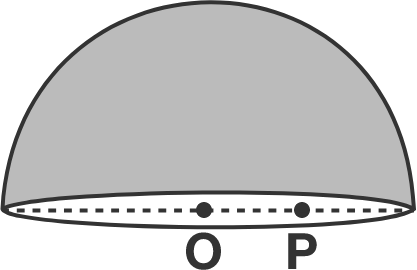A thin, non-conducting hemispherical shell contains a positive charge $Q$ on it, which is uniformly distributed on the shell. Point $\text{O}$ is the center of the base circle, and point $\text{P}$ is on a diameter of the base circle some distance away from $\text{O}.$

Which of the vectors shown below represents the direction of the electric field at point $\text{P}?$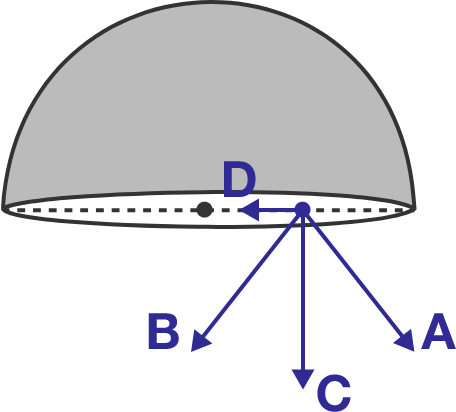Fred is an incredibly talented investor: so talented, in fact, that he doubles the amount of money in his bank account each year.

Fred doesn't worry about running out of money, so he spends increasing amounts every year, and never makes any deposits other than his original deposit. At the beginning of the first year (right after the original deposit), he spends $1. At the beginning of the second year, he spends$2. At the beginning of the third year, he spends $3, and so on. What is the minimum amount of money (in dollars) Fred needs in his original deposit so that he never runs out of money? Note: As an explicit example, this is what would happen if the initial deposit was$20:

$\begin{array}{|c|c|c|} \hline & \text{Account at the} & & \text{Account after} & \text{Account after} \\ \text{Year} & \text{beginning of year} & \text{Amount spent} & \text{spending} & \text{doubling} \\ \hline 1 & \20 & \1 & \19 & \38 \\ 2 & \38 & \2 & \36 & \72 \\ 3 & \72 & \3 & \69 & \138 \\ 4 & \138 & \4 & \134 & \268 \\ \hline \end{array}$

When 31513 and 34369 are each divided by a certain three-digit number, the remainders are equal. Find this remainder.

True or False?

For positive reals $a,$ $b,$ and $c,$ if $3abc = a^3 + b^3 + c^3,$ then it is necessarily true that $a=b=c.$


Note: One can interpret this geometrically. Consider a cuboid with side lengths $a,b,c,$ and three cubes with respective side lengths $a,b,c.$ If the sum of the volumes of the cubes is the same as three times the volume of the cuboid, then is the cuboid necessarily a cube?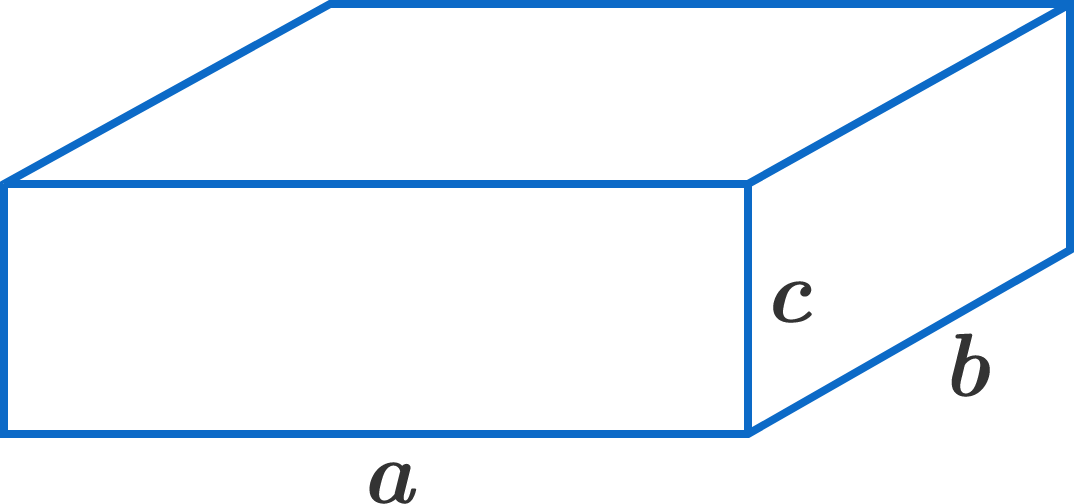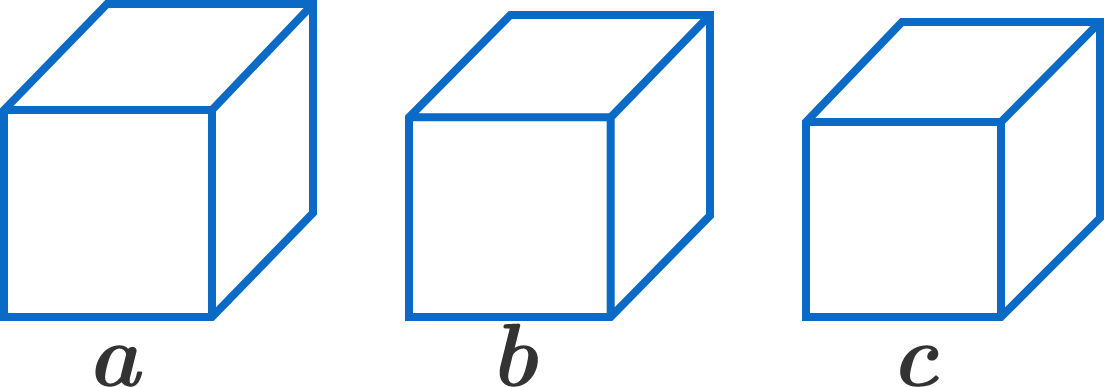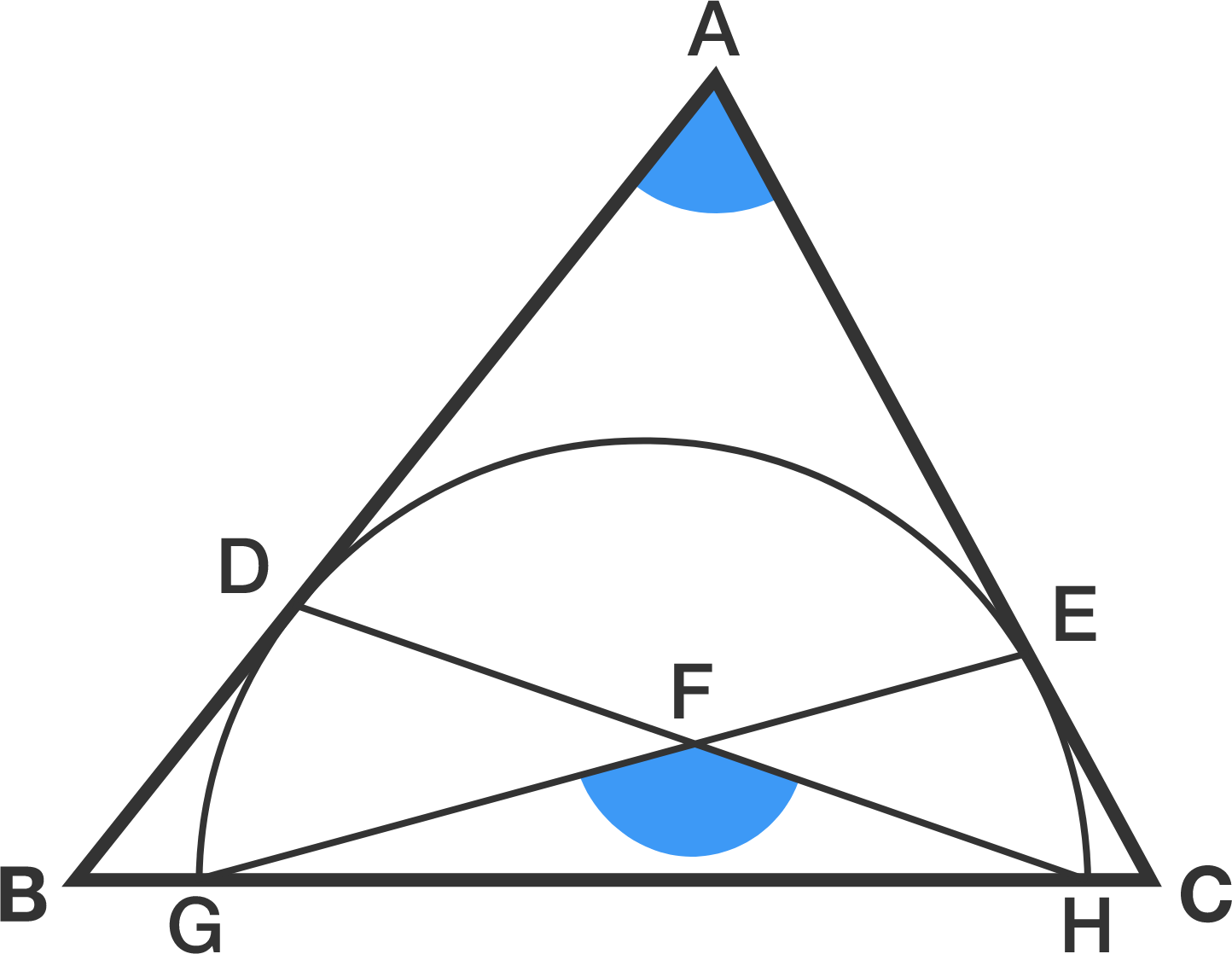As shown in the diagram, triangle $ABC$ inscribes a semi-circle such that $AB$ and $AC$ are tangents at points $D$ and $E,$ respectively.

$GH$ is the diameter of the semi-circle, and point $F$ is the intersection of $DH$ and $EG.$

If the 2 angles highlighted in blue are equal, find that angle in degrees.


Note: The diagram is not drawn to scale.

×